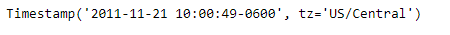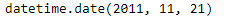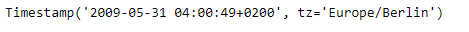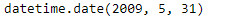# Python | Pandas Timestamp.date

• Last Updated : 08 Jan, 2019

Python is a great language for doing data analysis, primarily because of the fantastic ecosystem of data-centric python packages. Pandas is one of those packages and makes importing and analyzing data much easier.

Pandas` Timestamp.date()` function return a datetime object with same year, month and day as that of the given Timestamp object.

Attention geek! Strengthen your foundations with the Python Programming Foundation Course and learn the basics.

To begin with, your interview preparations Enhance your Data Structures concepts with the Python DS Course. And to begin with your Machine Learning Journey, join the Machine Learning - Basic Level Course

Syntax : Timestamp.date()

Parameters : None

Return : date

Example #1: Use `Timestamp.date()` function to return the date of the given Timestamp object as a datetime object.

 `# importing pandas as pd``import` `pandas as pd`` ` `# Create the Timestamp object``ts ``=` `pd.Timestamp(year ``=` `2011``,  month ``=` `11``, day ``=` `21``,``           ``hour ``=` `10``, second ``=` `49``, tz ``=` `'US/Central'``)`` ` `# Print the Timestamp object``print``(ts)`

Output :Now we will use the `Timestamp.date()` function to return the date as a datetime object.

 `# return as a datetime object ``ts.date()`

Output :As we can see in the output, the `Timestamp.date()` function has returned a datetime object containing the same year, month and day as that of the given Timestamp object.

Example #2: Use `Timestamp.date()` function to return the date of the given Timestamp object as a datetime object.

 `# importing pandas as pd``import` `pandas as pd`` ` `# Create the Timestamp object``ts ``=` `pd.Timestamp(year ``=` `2009``,  month ``=` `5``, day ``=` `31``,``        ``hour ``=` `4``, second ``=` `49``, tz ``=` `'Europe/Berlin'``)`` ` `# Print the Timestamp object``print``(ts)`

Output :Now we will use the `Timestamp.date()` function to return the date as a datetime object.

 `# return as a datetime object ``ts.date()`

Output :As we can see in the output, the `Timestamp.date()` function has returned a datetime object containing the same year, month and day as that of the given Timestamp object.

My Personal Notes arrow_drop_up